NEET  >  Vector Product of Two Vectors

# Vector Product of Two Vectors - Notes | Study Physics Class 11 - NEET

 1 Crore+ students have signed up on EduRev. Have you?

The cross product, area product or the vector product of two vectors is a binary operation on two vectors in three-dimensional spaces. It is denoted by ×. The cross product of two vectors is a vector. Let us consider two vectors denoted as. Let the product (also a vector) of these two vectors be denoted as.

Magnitude of the vector product

The magnitude of the vector product is given as,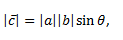Where a and b are the magnitudes of the vector and Ɵ is the angle between these two vectors. From the figure, we can see that there are two angles between any two vectors, that is, Ɵ and (360° – Ɵ). In this rule, we always consider the smaller angle that is less than 180°.

Direction of the vector product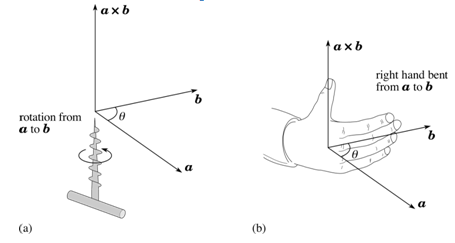The right-hand thumb rule is used in which we curl up the fingers of right hand around a line perpendicular to the plane of the vectors a and b and the curl the fingers in the direction from a to b, then the stretched thumb points in the direction of c.

Commutative property

Unlike the scalar product, cross product of two vectors is not commutative in nature. Mathematically, for scalar products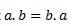But for vector products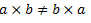As we know, the magnitude of both the cross products a × b and b × a is the same and is given by absinθ; but the curling of the right-hand fingers in case of a × b is from a to b, whereas in case of (b × a) it is from b to a, as per which, the two vectors are in opposite directions.
Mathematically,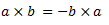Distributive property

Like the scalar product, vector product of two vectors is also distributive with respect to vector addition. Mathematically,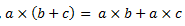In order to deal with the vector product of any two vectors, we need to know the vector product of two elementary vectors.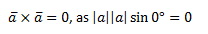Similarly, for the unit vectors following results hold good,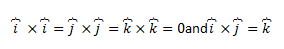The document Vector Product of Two Vectors - Notes | Study Physics Class 11 - NEET is a part of the NEET Course Physics Class 11.
All you need of NEET at this link: NEET

## Physics Class 11

127 videos|464 docs|210 tests
 Use Code STAYHOME200 and get INR 200 additional OFF

## Physics Class 11

127 videos|464 docs|210 tests

### How to Prepare for NEET

Read our guide to prepare for NEET which is created by Toppers & the best Teachers

Track your progress, build streaks, highlight & save important lessons and more!

,

,

,

,

,

,

,

,

,

,

,

,

,

,

,

,

,

,

,

,

,

;